# o 1.9 m, and a thickness of 0.76 cm. Find the energy transferred through the window...o 1.9 m, and a thickness of 0.76 cm. Find the energy transferred through the window by conduction in 12 hours on a day when the temperature of the house is 22*C and the outside temperature is 20.С. Take surface air layers into consideration. Neod Help? Lmn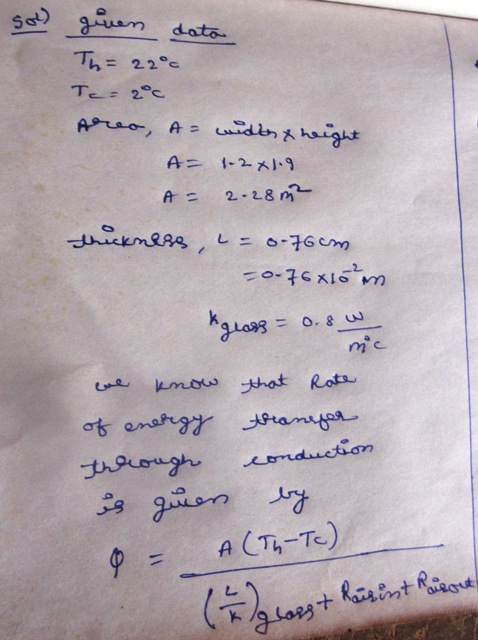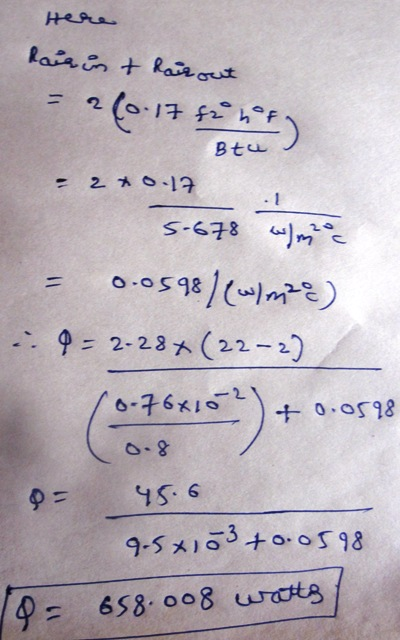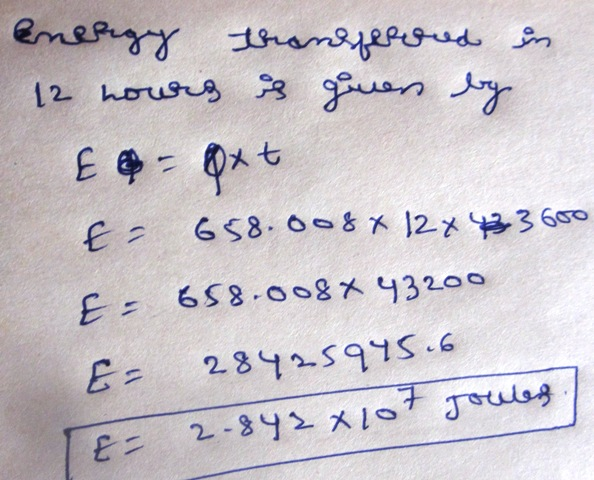##### Add Answer to: o 1.9 m, and a thickness of 0.76 cm. Find the energy transferred through the window...
Similar Homework Help Questions
• ### A rectangular glass window pane on a house has a width of 1.3 m, a height...

A rectangular glass window pane on a house has a width of 1.3 m, a height of 2.2 m, and a thickness of 0.42 cm. Find the energy transferred through the window by conduction in 12 hours on a day when the inside temperature of the house is 22°C and the outside temperature is 2.0°C. Take surface air layers into consideration. Answer in J.

• ### A rectangular glass window pane on a house has a width of 1.1 m, a height...

A rectangular glass window pane on a house has a width of 1.1 m, a height of 2.2 m, and a thickness of 0.65 cm. Find the energy transferred through the window by conduction in 12 hours on a day when the inside temperature of the house is 22°C and the outside temperature is 2.0°C. Take surface air layers into consideration. (Use 0.8 J/s · m · °C for the thermal conductivity of glass and 0.17 ft2 · °F ·...

• ### You drop an ice cube into an insulated container full of water and wait for the...

You drop an ice cube into an insulated container full of water and wait for the ice cube to completely melt. The ice cube initially has a mass of 80.0 g and a temperature of 0°C. The water (before the ice cube is added) has a mass of 660 g and an initial temperature of 20.0°C. What is the final temperature (in °C) of the mixture? (Assume no energy is lost to the walls of the container, or to the...

• ### (a) What is the rate of energy loss in watts per square meter through a glass...

(a) What is the rate of energy loss in watts per square meter through a glass window 3.5 mm thick if the outside temperature is -22°F and the inside temperature is +73°F? (b) A storm window having the same thickness of glass is installed parallel to the first window, with an air gap of 5.5 cm between the two windows. What now is the rate of energy loss if conduction is the only important energy-loss mechanism? (The thermal conductivity of...

• ### An energy technician is investigating energy loss through windows. The windowpane of interest is 0.600 cm...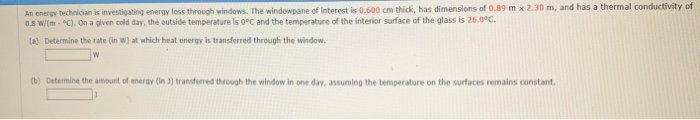An energy technician is investigating energy loss through windows. The windowpane of interest is 0.600 cm thick, has dimensions of 0.89 m x 2.30 m, and has a thermal conductivity of 0.8 wm-C). 0n a given cold day, the outside temperature is 0°C and the temperature of the interior surface of the glass is 26.0°c. (a) Determine the rate (in W) at which heat energy is transferred through the window. b) Determine the amount of energy (in 1) transferred through...

• ### (a) What is the rate of energy loss in watts per square meter through a glass...

(a) What is the rate of energy loss in watts per square meter through a glass window 2.2 mm thick if the outside temperature is -20°F and the inside temperature is +80°F? (b) A storm window having the same thickness of glass is installed parallel to the first window, with an air gap of 6.9 cm between the two windows. What now is the rate of energy loss if conduction is the only important energy-loss mechanism? (The thermal conductivity of...

• ### (a) Calculate the rate of heat conduction (in W) through a double-paned window that has a...

(a) Calculate the rate of heat conduction (in W) through a double-paned window that has a 5.00 m2 area and is made of two panes of 0.700 cm thick glass separated by a 1.00 cm air gap. The inside surface temperature is 17.0°C, while that on the outside is −20.0°C. (Hint: There are identical temperature drops across the two glass panes. First find these and then the temperature drop across the air gap. This problem ignores the increased heat transfer...

• ### (a) Calculate the rate of heat conduction (in w) through a double-paned window that has a...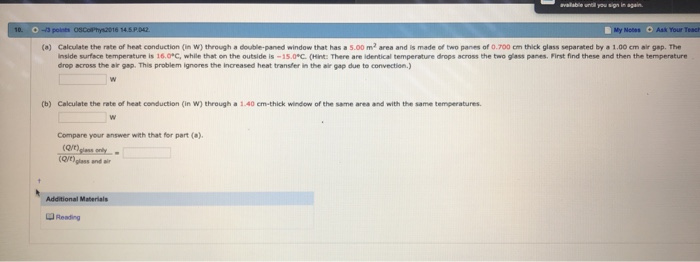(a) Calculate the rate of heat conduction (in w) through a double-paned window that has a s.00 m2 area and is made of two panes of 0.700 cm thick glass separated by a 1.00 cm air gap. The nside surface temperature is 16.0*C, while that on the outside is-1S.0 C (Hnt: There are identical temperature drops across the two glass panes. First find these and then the temperature drop across the ar gap. This problem ignores the increased heat transfer...

• ### Heat conduction occurs through any material, represented here by a rectangular bar, whether window glass or...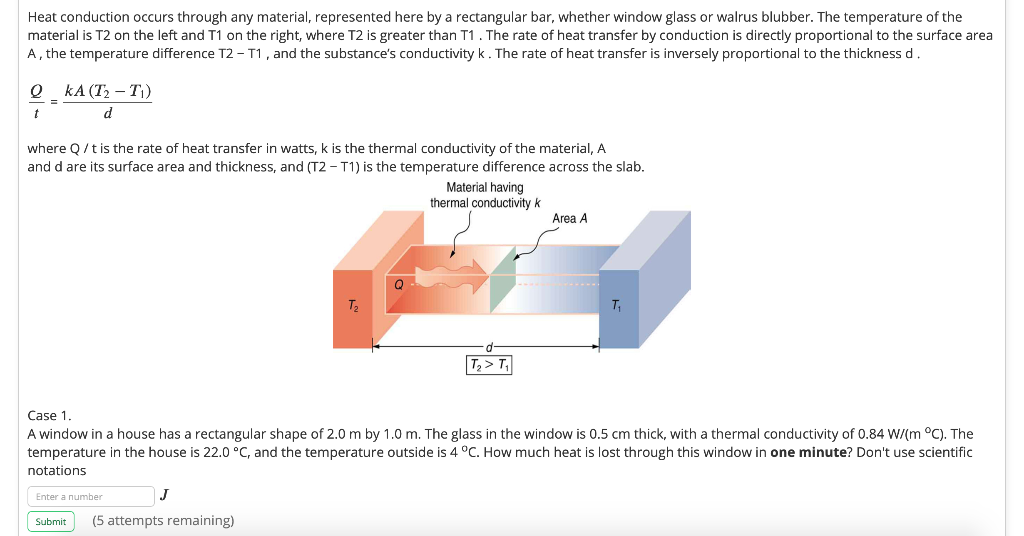Heat conduction occurs through any material, represented here by a rectangular bar, whether window glass or walrus blubber. The temperature of the material is T2 on the left and T1 on the right, where T2 is greater than T1. The rate of heat transfer by conduction is directly proportional to the surface area A, the temperature difference T2 - T1, and the substance's conductivity k. The rate of heat transfer is inversely proportional to the thickness d. Q kA (T2-T)...

• ### Consider the rate of heat conduction through a double-paned window that has a 1.45-m2 area and...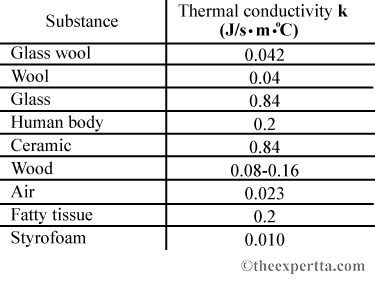Consider the rate of heat conduction through a double-paned window that has a 1.45-m2 area and is made of two panes of 0.715-cm-thick glass separated by a 1.25-cm air gap. You can ignore the increased heat transfer in the air gap due to convection. Calculate the rate of heat conduction through this window, in watts, given that the inside surface temperature is 15.0°C, while the outside temperature is -10.0°C. Make the assumption that the temperature differences across the two glass...

Free Homework App